# Classifying pediatric IBD stool samples (work in progress)¶

This notebook is a recoding of the analysis used in the PLoSONE paper: Non-Invasive Mapping of the Gastrointestinal Microbiota Identifies Children with Inflammatory Bowel Disease using python, sklearn and pandas.

We decided that the SLiME package, as it was packaged for the publication of the paper, should not be available anymore. This notebook replaces it, replicating the analysis executed on the paper with more up-to-date tools and (hopefully soon) expanding on its conclusion. I hope this can be the starting point for others trying to follow the same approach and improve upon it.

In :
%matplotlib inline

In :
import pandas as pd
import numpy as np
import matplotlib.pyplot as plt
from scipy import interp
from sklearn.ensemble import RandomForestClassifier
from sklearn.multiclass import OneVsRestClassifier
from sklearn.cross_validation import cross_val_score, StratifiedKFold, train_test_split, KFold
from sklearn.metrics import roc_curve, roc_auc_score, auc
from sklearn.preprocessing import LabelEncoder, label_binarize


The data came from two rounds of 16S sequencing of previously collected stool samples. Here we will use the OTU tables directly, which were created by using the RDP classifier and were subsequently normalized (details in the paper's methods).

Sequencing was performed at the Broad Institute. The first round of sequencing was dubbed CHIMP (Children Hospital IBD Pediatric), while the second round of sequencing -- performed following the request of an anonymous peer reviewer -- was termed 'blind validation'. Its purpose was to further validate the algorithm trained on the CHIMP dataset, as the reviewer did not think sufficient a "leave 20% out" approach on CHIMP was sufficient to demonstrate robust prediction. These were used as training and test set in the last figure of the paper respectively.

It is useful to join the two data sets here.

In :
#get the CHIMP training data

#just make sure the labels are the same
X_chimp.sort_index(inplace=True)
y_chimp.sort_index(inplace=True)
assert (X_chimp.index == y_chimp.index).all()

In :
## do the same for the blind validation test data
delimiter="\t", index_col=0)
index_col=0)

X_blind.sort_index(inplace=True)
y_blind.sort_index(inplace=True)
assert (X_blind.index == y_blind.index).all()

In :
#concatenate using pandas
X = pd.concat([X_chimp, X_blind], keys=['chimp','blind'])

Out:
cls_Actinobacteria cls_Alphaproteobacteria cls_Bacilli cls_Bacteroidia cls_Betaproteobacteria cls_Clostridia cls_Cyanobacteria cls_Deltaproteobacteria cls_Epsilonproteobacteria cls_Erysipelotrichi ... phylum_Euryarchaeota phylum_Firmicutes phylum_Fusobacteria phylum_Lentisphaerae phylum_NA phylum_Proteobacteria phylum_Spirochaetes phylum_Synergistetes phylum_TM7 phylum_Verrucomicrobia
chimp 003A 0.000000 0.000549 0.014827 0.002197 0.000275 0.230917 0 0.000000 0 0.000000 ... 0 0.245744 0.023064 0 0.000000 0.728995 0 NaN NaN 0
004A 0.000000 0.000000 0.002486 0.754195 0.000000 0.230889 0 0.000932 0 0.001865 ... 0 0.238036 0.000000 0 0.000000 0.007147 0 NaN NaN 0
005A 0.006521 0.000000 0.026084 0.000000 0.000000 0.908706 0 0.000000 0 0.054451 ... 0 0.990545 0.000000 0 0.000326 0.002608 0 NaN NaN 0
008A 0.000315 0.000000 0.000210 0.837024 0.035995 0.112499 0 0.000000 0 0.000840 ... 0 0.113758 0.000000 0 0.000000 0.048903 0 NaN NaN 0
009A 0.001291 0.000000 0.001550 0.823864 0.024277 0.118027 0 0.000000 0 0.000000 ... 0 0.119576 0.000000 0 0.000000 0.055269 0 NaN NaN 0

5 rows × 284 columns

In :
X.fillna(value=0,inplace=True) #replace NAs with zeroes

In :
y_dx = pd.concat([y_chimp.dx, y_blind.dx], keys=['chimp','blind'])
y_dx #btw, what joy is to use pandas over R/dplyr for this. so intuitive and fast.

Out:
       sample
chimp  003A      UC
004A      UC
005A      NM
008A      CD
009A      UC
012A      UC
016A      CD
018A      CD
024A      NM
025A      CD
026A      UC
027A      UC
030A      NM
031A      NM
034B      UC
...
blind  251-AX    CD
252-AX    CD
253-AZ    UC
254-AX    CD
255-AZ    UC
256-AX    CD
258-AZ    CD
259-AX    NM
260-AY    CD
261-AX    UC
262-AZ    CD
263-AX    UC
264-AX    NM
265-AX    UC
291-AX    CD
Name: dx, Length: 158, dtype: object
In :
#convert the training and testing labels to numerical values
le = LabelEncoder()
le.fit(y_dx)
y = le.transform(y_dx)

# just for reference, the columns of the binarized label read respectively:
le.inverse_transform([0,1,2])

Out:
array(['CD', 'NM', 'UC'], dtype=object)

## Label classification¶

Please note that the ROC plots will look different everytime the notebook is run due to the random nature of the cross-validation split

### Vanilla classifier¶

We will go straight to using RandomForest and a 10-fold cross validation. Many other models were tried but RandomForest consistently prevented overfitting. First let's get an idea of how it looks like when you try to classify all the labels at the same time.

In :
clf = RandomForestClassifier(n_estimators=50, oob_score=True)
clf.fit(X.values, y)
scores = cross_val_score(clf, X.values, y, cv=10)

print("Cross validation score:")
print(scores.mean())

importances = clf.feature_importances_
std = np.std([tree.feature_importances_ for tree in clf.estimators_],axis = 0)
indices = np.argsort(importances)[::-1]

print("feature ranking:")

for f in range(20):
print("%d. feature %d (%f)" % (f + 1, indices[f], importances[indices[f]]))

Cross validation score:
0.567471988796
feature ranking:
1. feature 71 (0.030080)
2. feature 92 (0.029311)
3. feature 52 (0.021462)
4. feature 223 (0.019596)
5. feature 12 (0.018157)
6. feature 66 (0.017697)
7. feature 45 (0.017373)
8. feature 145 (0.016363)
9. feature 216 (0.015820)
10. feature 79 (0.015636)
11. feature 65 (0.014432)
12. feature 99 (0.014312)
13. feature 109 (0.014086)
14. feature 72 (0.014012)
15. feature 51 (0.013417)
16. feature 37 (0.013062)
17. feature 27 (0.013053)
18. feature 2 (0.012811)
19. feature 0 (0.012587)
20. feature 272 (0.012439)


### Build a One-vs-all ROC curve (not cross validated)¶

To build a ROC curve we need to binarize the variable and run the classifier as one class vs. all others

In :
y_bin = label_binarize(y,classes=[0,1,2])
n_classes = y_bin.shape

In :
X_train, X_test, y_train, y_test = train_test_split(X.values, y_bin, test_size=.3)

In :
clf1 = OneVsRestClassifier(RandomForestClassifier(n_estimators=50))
y_score = clf1.fit(X_train, y_train).predict_proba(X_test)

/usr/local/lib/python3.4/site-packages/sklearn/utils/__init__.py:93: DeprecationWarning: Function multilabel_ is deprecated; Attribute multilabel_ is deprecated and will be removed in 0.17. Use 'y_type_.startswith('multilabel')' instead
warnings.warn(msg, category=DeprecationWarning)


The probabilities of each class are now in a numpy array where each row corresponds to sample and each column to the label in question (CD, NM or UC). Let's take a pick at the first 10:

In :
y_score[:10,:]

Out:
array([[ 0.48,  0.1 ,  0.34],
[ 0.56,  0.08,  0.56],
[ 0.02,  0.74,  0.14],
[ 0.32,  0.16,  0.58],
[ 0.16,  0.4 ,  0.58],
[ 0.28,  0.02,  0.76],
[ 0.34,  0.42,  0.34],
[ 0.48,  0.06,  0.58],
[ 0.3 ,  0.08,  0.48],
[ 0.28,  0.64,  0.2 ]])
In :
# Compute ROC curve and ROC area for each class
fpr = dict()
tpr = dict()
roc_auc = dict()
for i in range(n_classes):
fpr[i], tpr[i], _ = roc_curve(y_test[:, i], y_score[:, i])
roc_auc[i] = roc_auc_score(y_test[:,i], y_score[:,i],average="micro")

In :
# Plot of a ROC curve for a specific class
plt.figure()
plt.plot(fpr, tpr, label='ROC curve (area = %0.2f)' % roc_auc)
plt.plot([0, 1], [0, 1], 'k--')
plt.xlim([0.0, 1.0])
plt.ylim([0.0, 1.05])
plt.xlabel('False Positive Rate')
plt.ylabel('True Positive Rate')
plt.title('ROC - UC vs all')
plt.legend(loc="lower right")
plt.show()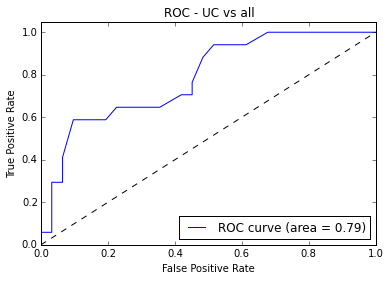In :
# Plot ROC curves all together now
plt.figure()

for i in range(n_classes):
plt.plot(fpr[i], tpr[i], label='ROC curve {0} (area = {1:0.2f})'
''.format(le.inverse_transform(i), roc_auc[i]))

plt.plot([0, 1], [0, 1], 'k--')
plt.xlim([0.0, 1.0])
plt.ylim([0.0, 1.05])
plt.xlabel('False Positive Rate')
plt.ylabel('True Positive Rate')
plt.title('ROC - one random 1/3 split')
plt.legend(loc="lower right")
plt.show()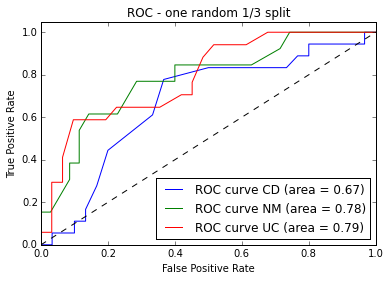### ROC cross validated Figure 6A¶

#### top panel: one vs all¶

In :
# Run classifier with cross-validation and plot ROC curves

for dx in range(n_classes):
cv = StratifiedKFold(y_bin[:,dx], n_folds=10)
classifier = RandomForestClassifier()

mean_tpr = 0.0
mean_fpr = np.linspace(0, 1, 100)
all_tpr = []

for i, (train, test) in enumerate(cv):
probas_ = classifier.fit(X.iloc[train], y_bin[train,dx]).predict_proba(X.iloc[test])
# Compute ROC curve and area the curve
fpr, tpr, thresholds = roc_curve(y_bin[test,dx], probas_[:, 1])
mean_tpr += interp(mean_fpr, fpr, tpr)
mean_tpr = 0.0
roc_auc = auc(fpr, tpr)
#plt.plot(fpr, tpr, lw=1, label='ROC fold %d (area = %0.2f)' % (i, roc_auc))

mean_tpr /= len(cv)
mean_tpr[-1] = 1.0
mean_auc = auc(mean_fpr, mean_tpr)
plt.plot(mean_fpr, mean_tpr,
label='Mean ROC %s (area = %0.2f)' % (le.inverse_transform(dx), mean_auc), lw=1)

plt.xlim([-0.05, 1.05])
plt.ylim([-0.05, 1.05])
plt.xlabel('False Positive Rate')
plt.ylabel('True Positive Rate')
plt.title('Figure 6A - ROC - crossvalidated - one vs. all')
plt.legend(loc="lower right")
plt.show()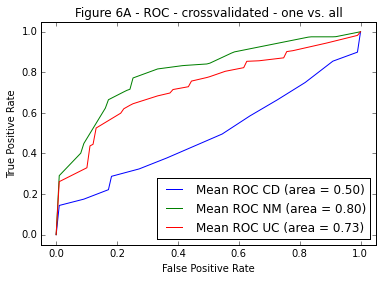#### bottom panel: CD vs UC¶

In :
# Only CD vs UC

X_cduc = X[(y == 0) | (y == 2)]
y_cduc = y[(y == 0) | (y == 2)]
np.place(y_cduc,y_cduc == 2, 1)

cv = StratifiedKFold(y_cduc, n_folds=10)
clf_cduc = RandomForestClassifier()

mean_tpr = 0.0
mean_fpr = np.linspace(0, 1, 100)
all_tpr = []

for i, (train, test) in enumerate(cv):
fitted = classifier.fit(X_cduc.iloc[train], y_cduc[train])
probas_ = fitted.predict_proba(X_cduc.iloc[test])
scored_ = fitted.predict(X_cduc.iloc[test])
# Compute ROC curve and area the curve
fpr, tpr, thresholds = roc_curve(y_cduc[test], probas_[:, 1])
mean_tpr += interp(mean_fpr, fpr, tpr)
mean_tpr = 0.0
#roc_auc = auc(fpr, tpr)
roc_auc = roc_auc_score(scored_, y_cduc[test], average="micro")
#plt.plot(fpr, tpr, lw=1, label='ROC fold %d (area = %0.2f)' % (i, roc_auc))

mean_tpr /= len(cv)
mean_tpr[-1] = 1.0
mean_auc = auc(mean_fpr, mean_tpr)
plt.plot(mean_fpr, mean_tpr,
label='Mean ROC (area = %0.2f)' % mean_auc, lw=1)

plt.xlim([-0.05, 1.05])
plt.ylim([-0.05, 1.05])
plt.xlabel('False Positive Rate')
plt.ylabel('True Positive Rate')
plt.title('Figure 6A - ROC - crossvalidated - CD vs UC')
plt.legend(loc="lower right")
plt.show()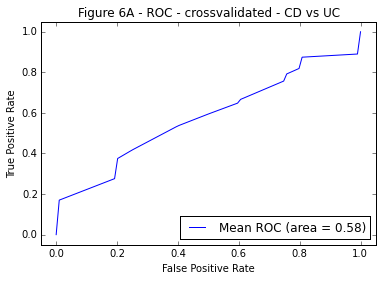## Comparison with the Frank et al. dataset¶

The original paper links to the available dataset. As outlined in the paper, the SLiME pipeline runs RDP on the fasta files and outputs a matrix of normalized abundance of each family, class, genus, etc.

I am going to load directly that table, which has had the ibd classification label appended to it.

In :
frank = pd.read_csv('data/pace/pace.rdpout.xtab.norm.ibd.csv')
frank_label = frank['ibd']
del frank['ibd']
frank_label = (frank_label == 'yes')
frank_label.value_counts()

Out:
True     129
False     61
dtype: int64
In :
def crossval_roc(X, y):
cv = StratifiedKFold(y, n_folds=10)
clf = RandomForestClassifier()

mean_tpr = 0.0
mean_fpr = np.linspace(0, 1, 100)
all_tpr = []

for i, (train, test) in enumerate(cv):
fitted = clf.fit(X[train], y[train])
probas_ = fitted.predict_proba(X[test])
scored_ = fitted.predict(X[test])
# Compute ROC curve and area the curve
fpr, tpr, thresholds = roc_curve(y[test], probas_[:, 1])
mean_tpr += interp(mean_fpr, fpr, tpr)
mean_tpr = 0.0
#roc_auc = auc(fpr, tpr)
roc_auc = roc_auc_score(scored_, y[test], average="micro")
#plt.plot(fpr, tpr, lw=1, label='ROC fold %d (area = %0.2f)' % (i, roc_auc))

mean_tpr /= len(cv)
mean_tpr[-1] = 1.0
mean_auc = auc(mean_fpr, mean_tpr)
return plt.plot(mean_fpr, mean_tpr,
label='Mean ROC (area = %0.2f)' % mean_auc, lw=1)

fig = crossval_roc(frank.values,frank_label.values)
plt.xlim([-0.05, 1.05])
plt.ylim([-0.05, 1.05])
plt.xlabel('False Positive Rate')
plt.ylabel('True Positive Rate')
plt.title('Frank et al. cross-validated ROC')
plt.legend(loc="lower right")
plt.show()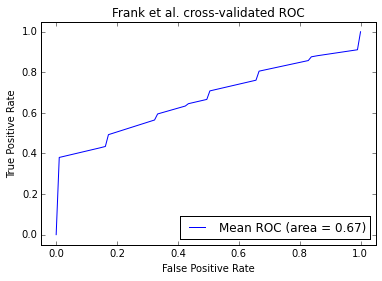In :


Out:
array([False,  True], dtype=bool)
In [ ]: